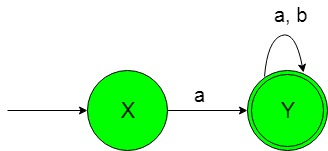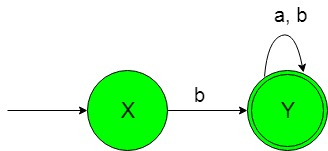# Designing Non-Deterministic Finite Automata (Set 1)

Prerequisite: Finite Automata Introduction
In this article, we will see some designing of Non-Deterministic Finite Automata (NFA).

Problem-1: Construction of a minimal NFA accepting a set of strings over {a, b} in which each string of the language starts with ‘a’.
Explanation: The desired language will be like:

`L1 = {ab, abba, abaa, ...........}`

Here as we can see that each string of the above language starts with ‘a’ and end with any alphabet either ‘a’ or ‘b’.
But the below language is not accepted by this NFA because none of the string of below language starts with ‘a’.

`L2 = {ba, ba, babaaa..............}`

The state transition diagram of the desired language will be like below:In the above NFA, the initial state ‘X’ on getting ‘a’ as the input it transits to a final state ‘Y’. The final state ‘Y’ on getting either ‘a’ or ‘b’ as the input it remains in the state of itself.

### Python Implementation:

 `def` `stateX(n): ` `    ``#if length of n become 0  ` `    ``#then print not accepted ` `    ``if``(``len``(n)``=``=``0``): ` `        ``print``(``"string not accepted"``) ` `         `  `    ``else``:  ` `        ``#if at zero index  ` `        ``#'a' found call ` `        ``#stateY function     ` `        ``if` `(n[``0``]``=``=``'a'``): ` `            ``stateY(n[``1``:]) ` `         `  `        ``#if at zero index  ` `        ``#'b' then print  ` `        ``#not accepted ` `        ``elif` `(n[``0``]``=``=``'b'``): ` `            ``print``(``"string not accepted"``)    ` `        `  `def` `stateY(n): ` `    ``#if length of n become 0  ` `    ``#then print accepted ` `    ``if``(``len``(n)``=``=``0``): ` `        ``print``(``"string accepted"``) ` `         `  `    ``else``:   ` `        ``#if at zero index  ` `        ``#'a' found call ` `        ``#stateY function     ` `        ``if` `(n[``0``]``=``=``'a'``): ` `            ``stateY(n[``1``:]) ` `             `  `        ``#if at zero index  ` `        ``#'b' found call ` `        ``#stateY function     ` `        ``elif` `(n[``0``]``=``=``'b'``): ` `            ``stateY(n[``1``:])     ` `         `  ` `  `             `  `             `  `#take input ` `n``=``input``() ` ` `  `#call stateA function ` `#to check the input ` `stateX(n) `

Problem-2: Construction of a minimal NFA accepting a set of strings over {a, b} in which each string of the language is not starting with ‘a’.
Explanation: The desired language will be like:

`L1 = {ba, bba, bbaa, ...........}`

Here as we can see that each string of the above language is not starting with ‘a’ but can end with either ‘a’ or ‘b’.
But the below language is not accepted by this NFA because some of the string of below language starts with ‘a’.

`L2 = {ab, aba, ababaab..............}`

The state transition diagram of the desired language will be like below:In the above NFA, the initial state ‘X’ on getting ‘b’ as the input it transits to a final state ‘Y’. The final state ‘Y’ on getting either ‘a’ or ‘b’ as the input it remains in the state of itself.

### Python Implementation:

 `def` `stateX(n): ` `    ``#if length of n become 0  ` `    ``#then print not accepted ` `    ``if``(``len``(n)``=``=``0``): ` `        ``print``(``"string not accepted"``) ` `         `  `    ``else``:  ` `        ``#if at zero index  ` `        ``#'b' found call ` `        ``#stateY function     ` `        ``if` `(n[``0``]``=``=``'b'``): ` `            ``stateY(n[``1``:]) ` `         `  `        ``#if at zero index  ` `        ``#'a' then print  ` `        ``#not accepted ` `        ``elif` `(n[``0``]``=``=``'a'``): ` `            ``print``(``"string not accepted"``)    ` `        `  `def` `stateY(n): ` `    ``#if length of n become 0  ` `    ``#then print accepted ` `    ``if``(``len``(n)``=``=``0``): ` `        ``print``(``"string accepted"``) ` `         `  `    ``else``:   ` `        ``#if at zero index  ` `        ``#'a' found call ` `        ``#stateY function     ` `        ``if` `(n[``0``]``=``=``'a'``): ` `            ``stateY(n[``1``:]) ` `             `  `        ``#if at zero index  ` `        ``#'b' found call ` `        ``#stateY function     ` `        ``elif` `(n[``0``]``=``=``'b'``): ` `            ``stateY(n[``1``:])     ` `         `  ` `  `             `  `             `  `#take input ` `n``=``input``() ` ` `  `#call stateA function ` `#to check the input ` `stateX(n) `

Attention reader! Don’t stop learning now. Get hold of all the important CS Theory concepts for SDE interviews with the CS Theory Course at a student-friendly price and become industry ready.

My Personal Notes arrow_drop_upCheck out this Author's contributed articles.

If you like GeeksforGeeks and would like to contribute, you can also write an article using contribute.geeksforgeeks.org or mail your article to contribute@geeksforgeeks.org. See your article appearing on the GeeksforGeeks main page and help other Geeks.

Please Improve this article if you find anything incorrect by clicking on the "Improve Article" button below.

Improved By : _mridul_bhardwaj_

Article Tags :

Be the First to upvote.

Please write to us at contribute@geeksforgeeks.org to report any issue with the above content.